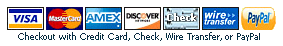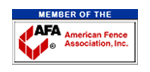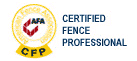# How Much Material is Needed to Fence an Acre of Land?

Many times customers want to know how much it is to fence an acre of land. An Acre is a measurement of area, whereas when you are figuring fence materials, you typically are dealing with finding the perimeter, or distance around a property. It is still pretty easy to figure the perimeter if you know the area of your property.

One acre of land comprises 43,560 square feet. If you assume that your property is 4 equal sides, then you can take the square root (√) of 43,560 and find out that each side would measure 209'. Since this is based on a square, you would take 209 linear feet x 4 sides, to arrive at 835 linear feet of fence to enclose that acre.

Example 1 - Figuring Amount of Fence to Enclose 1 Acre of Land:

1) One Acre = 43,560 square feet
2) To find the length of one side you need the square root (√) of 43,560 which equals 209 linear feet
3) 209 (linear feet) x 4 (sides) = 836 linear feet to enclose 1 Acres.

Most people don't have an exact acre so if you if you want to figure multiple acres, multiply 43,560 times the number of acres, then take the square root of that product, and multiply it times 4 which will give you the total footage of fence required.

Example 2 - Figuring Amount of Fence to Enclose 10 Acres of Land:

1) One Acre = 43,560 square feet
2) Ten Acres = 10 x 43,560 square feet - or - 435,600 square feet
3) To find the length of one side you need the square root (√) of 435,600 which equals 660 linear feet
4) 660 (linear feet) x 4 (sides) = 2,640 linear feet to enclose 10 Acres.

Likewise, many people don't even have one acre. In that instance you could take the fraction of an acre that you have and follow the steps above to find the total linear footage required to fence your property.

Example 3 - Figuring Amount of Fence to Enclose 1/4 of an Acre of Land:

1) One Acre = 43,560 square feet
2) 1/4 Acre = .25 x 43,560 square feet - or - 10,890 square feet
3) To find the length of one side you need the square root (√) of 10,890 which equals 105 linear feet
4) 104 (linear feet) x 4 (sides) = 420 linear feet to enclose a quarter of an acre.

With this new found information, it is easy to estimate the total cost of a fence to enclose your property. If for example, a 4' high residential chain link fence costs \$4.56 per linear foot, then your rough cost on the one acre would be \$3,812.16 in materials (\$4.56 x 836 linear feet), the 10 acres would be \$12,038.40 (\$4.56 x 2,640 linear feet), and if you are estimate the cost for a quarter of an acre, it would cost \$1,915.20 in materials (\$4.56 x 420 linear feet).

Please feel free to use the calculators below to find your estimated costs and material lists once you use the above formulas to find the total footage of fencing you need.